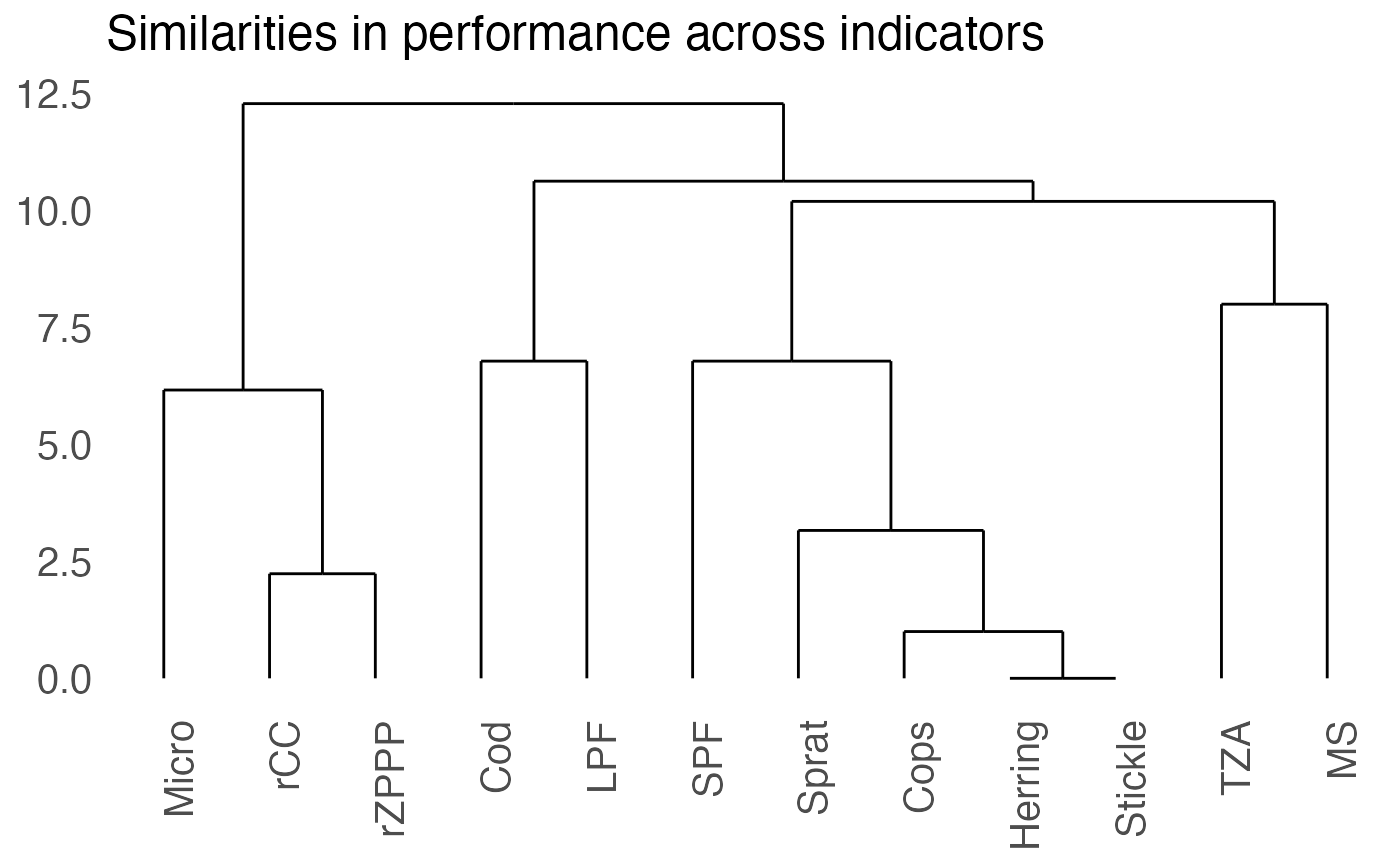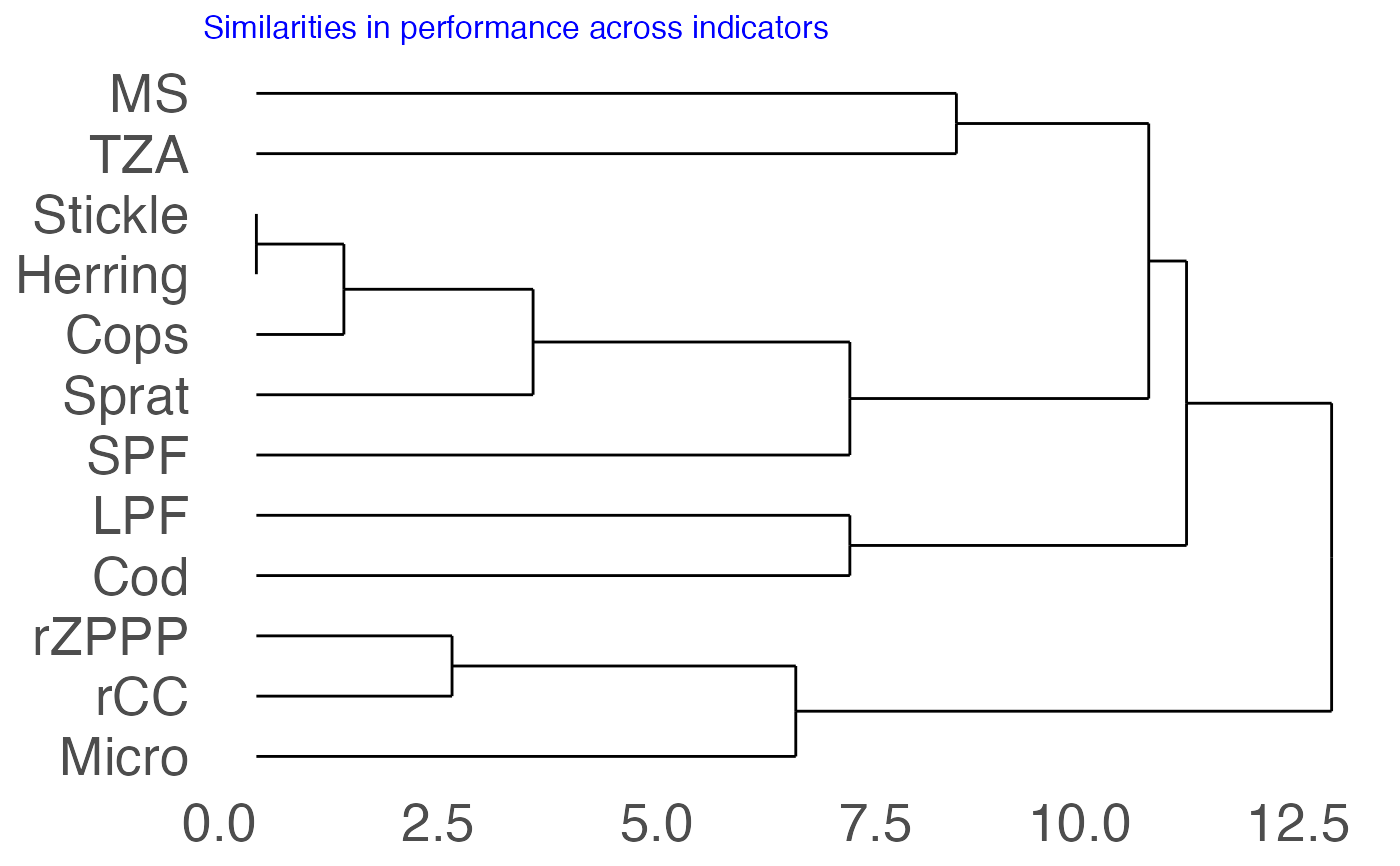plot_clust_sc generates from the cluster analysis a dendrogram based on the ggplot2 and ggdendro packages.

plot_clust_sc(x, rotate = FALSE, text_size = 15)

## Arguments

x Output from the cluster analysis (object of class hclust). If TRUE, rotates plot by 90 degrees. Size of the title and axes labels.

## Value

The function returns a ggplot object.

ggplot, dendro_data for the data extraction from the hclust object to produce the dendrogram

Other score-based IND performance functions: clust_sc(), dist_sc_group(), dist_sc(), expect_resp(), plot_spiechart(), scoring(), summary_sc()

## Examples

# Using the Baltic Sea demo data
scores_tbl <- scoring(trend_tbl = model_trend_ex,
mod_tbl = all_results_ex, press_type = press_type_ex)
scores_mat <- summary_sc(scores_tbl)\$scores_matrix
dist_matrix <- dist_sc(scores_mat, method_dist = "euclidean")
clust_analysis <- clust_sc(dist_matrix, method_clust = "complete")
#> With the selected agglomeration method (complete), the cophonetic correlation coeffient is 0.852 and the Gower distance is 481.34.plot_clust_sc(clust_analysis)# To modify the plot:
plot_clust_sc(clust_analysis, rotate = TRUE, text_size = 20) +
ggplot2::theme(title = ggplot2::element_text(colour = "blue", size = 10))